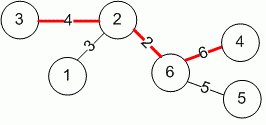Competitions

# Longest path in a tree

Undirected weighted tree is given. Find the length of the longest path. Find two vertices the distance between which is maximum.

#### Input

First line contains the number of vertices in the tree n (2n105). Next n - 1 lines describe the edges. Each line contains three integers: numbers of vertices connected with an edge (vertices are numbered from 1 to n) and the weight w (1w105) of an edge.

#### Output

Print the length of the longest path.Time limit 2 seconds
Memory limit 128 MiB
Input example #1
6
1 2 3
2 3 4
2 6 2
6 4 6
6 5 5

Output example #1
12